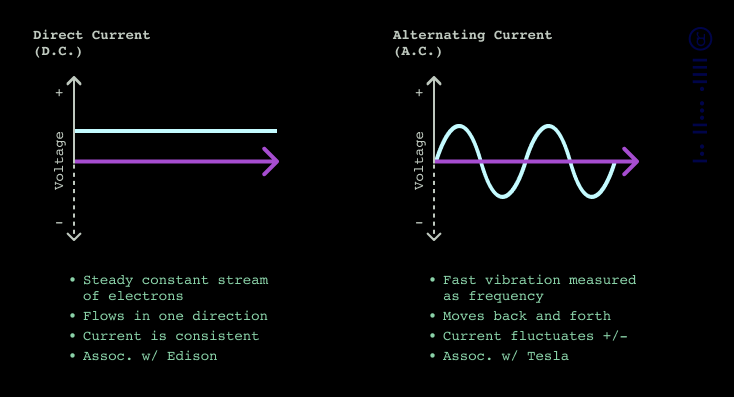<< index

by disinfoniacs #69 & #1

## ac/dc

>>>>

### tldr

• Current can flow in two ways: AC and DC
• AC current alternates its direction between positive and negative
• Frequency is the number of times per second that alternating current makes a complete cycle, and it's measured in Hertz
• Impedance is the opposition to AC current flow in a circuit, measured in ohms
• DC current flows in one direction and is used to power a great deal of ham radio equipment.

## in depthCurrent is the flow of charged particles, such as electrons, through a conductive medium like a wire. It is an essential concept in understanding how electrical circuits work, and it is measured in amperes or "amps." In a circuit, current can flow in two ways: alternating current (AC) or direct current (DC).

AC is the type of current used in home outlets and power grids. It alternates its direction between positive and negative at a specific rate, known as its frequency. The frequency is the number of times the current completes a cycle per second, and it is measured in Hertz (Hz). In the United States, the standard frequency of AC power is 60 Hz, meaning that the current alternates direction 60 times per second. In Europe and many other parts of the world, the standard frequency is 50 Hz.

AC has become the standard for power transmission and distribution because it allows for efficient power transfer over long distances. However, some electronics, particularly radio equipment, require DC power to operate.

Impedance is a critical concept in understanding electronic circuits, and it is a measure of how much the circuit resists the flow of alternating current. It's the resistance of the circuit to the changing direction of the current, which makes it distinct from direct current (DC) resistance. Impedance is an essential consideration in radio frequency (RF) systems, where the impedance of the transmitter and receiver must be matched for maximum power transfer.

Like resistance, impedance is measured in ohms, which is the unit of electrical resistance. Impedance can vary depending on the type of circuit and the frequency of the AC signal passing through it. In a circuit that has a high impedance, the current will be restricted, and the circuit will consume more power, which can cause problems in the circuit. On the other hand, a circuit with low impedance will have a higher current flow and consume less power.

Direct current (DC) is a type of electrical current that flows in one direction. Unlike AC, which alternates direction, DC always flows in a consistent direction, providing a steady and continuous stream of electrical energy. This type of current is commonly used to power electronic devices and equipment, including most ham radio gear. The power source for most portable ham radio equipment is a battery that provides DC power. DC is also used in a variety of other applications, including solar power systems, microprocessors, and electric vehicles, where a steady and reliable supply of power is essential. DC voltage levels are typically specified in volts, and current flow is measured in amperes. DC circuits also have their own set of unique characteristics, such as voltage drop, which occurs when current flows through a conductor, creating resistance and causing a drop in voltage. Understanding the properties and behaviors of DC current is essential for building and operating reliable electronic systems and devices.

---

<< index